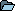ContinuousWave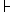WhalerModerated Discussion AreasContinuousWave: Small Boat ElectricalWire Conductor Size for Power Distribution
 Author Topic:   Wire Conductor Size for Power Distribution jimh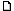posted 05-11-2013 01:00 PM ET (US)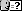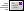Wire Conductor Size for Power DistributionIn distribution of power, there are two concerns in choosing the size of the conductors that will carry the electrical current:--the rating of the conductor for current capacity--the voltage drop that will occur in the conductor In systems where the distributed voltage is low, the concern for the voltage drop tends to limit the length of the conductor that can be used. This article examines this relationship in more detail.The resistance of a wire for a certain unit of length is a function of the material used for the wire and its cross section area. For a conductor made of copper and sized according to the American Wire Gauge (AWG standard) we can find the resistance per 1,000-feet of conductor from a table. Seefor a good listing of this data. If we use a wire of 10-AWG, we see that 1,000-feet of 10-AWG wire will have a resistance of 1-Ohm. This value is really a physical property of copper, so there is not much variance seen in listings of the resistance of copper wire.The current carrying capacity or current rating is a value that is more difficult to define with precision. As current flows through a conductor it encounters resistance, which results in a power loss. The power is converted to heat. The wire becomes warmer. The amount of temperature increase of the wire above the ambient temperature becomes a factor in rating the maximum current. If the conductor is a single conductor and is in free air space, much more heating can be tolerated. If the conductor is in a bundle with other conductors and enclosed in a conduit with little cooling air, the maximum current allowed will be lower. The type of insulation also affects the current rating. Expensive insulation material like Teflon can tolerate higher temperatures. The result of the many variables affecting wire temperature is the maximum current rating is more variable. For our example conductor of 10-AWG, we see the maximum current for a single conductor in free air is 55-Amperes. For other situations, such as power distribution, the maximum current is derated to only 15-Amperes.When a current flows in a wire, the voltage drop in the conductor will be equal to the resistance of the wire in Ohms times the current in the wire in Amperes. For wire of a particular gauge, we can compute the resistance by the length of the wire and the resistance of that wire gauge per 1,000-feet. We can say(1) VoltageDrop = Length-in-Feet x (Resistance/1,000-feet) x CurrentTypically in power distribution a limit on the voltage drop to a certain percentage of the system voltage is required. A limit of three-percent is often used. We can set this as our maximum allowed VoltageDrop byVoltageDrop = SystemVoltage x 0.03In the case of a 12-Volt system, the result is VoltageDrop = 0.36-VoltsNow we can calculate the Length-in-Feet of a particular conductor that will create a voltage drop of 0.36. We solve equation (1) for Length-in-Feet:(2) Length-in-Feet = VoltageDrop / [(Resistance/1,000-feet) x Current]This rearranges to(3) Length-in-Feet = VoltageDrop x 1000 / (Resistance x Current)Now we explore a 10-AWG conductor at its maximum rated current of 55-Amperes in a 12-Volt systemLength-in-Feet = 0.36-Volt / [1-Ohm/1,000-feet) x 55-Ampere]Length-in-Feet = 6.54-feetBecause this is the total length of the conductors, in a two-way circuit, the maximum distance that a 10-AWG conductor could carry its rated 55-Amperes before the voltage drop in a 12-Volt system exceeded 3-percent is only 3.25-feet.Now we explore a current of only 15-Amperes, which is the rated current for 10-AWG when used in power distribution (according to the table mentioned above). (See the footnotes in the table for the criteria used to obtain this rating.)Recalculating the Length-in-Feet for the 10-AWG conductor before we reach the maximum voltage drop of 3-percent in a 12-Volt system, we haveLength-in-Feet = (0.36-Volt x 1000) / (15-Ampere x 1-Ohm) = 24-feetAgain, for a two-way circuit, this value is divided in two, and we see that a 10-AWG conductor can be used to distribute 15-Amperes in a 12-Volt system for only a maximum distance of 12-feet.Let's examine a typical situation in a small boat with power distribution from a battery at the stern to a secondary power distribution center at the helm, a distance of about 15-feet. A conductor of 8-AWG is used. The resistance per 1,000-feet for 8-AWG is 0.6282-Ohms. The rated maximum current in power distribution is 24-Amperes. We find the maximum length fromLength-in-Feet = (0.36-Volt x 1000-feet/ (24-Ampere x 0.6282-Ohm) = 23.9-feetFor a two-way circuit, the maximum length is half that or about 12-feet.Let's try a 4-AWG conductor: the resistance per 1,000-feet is 0.2485 and the maximum current is 60-Amperes. The maximum length for 3-percent drop at 12-Volts is Length-in-Feet = (0.36-Volt x 1000-feet/ (60-Ampere x 0.0.2485-Ohm) = 24.1-feetFor a two-way circuit, the maximum length is half that or about 12-feet.I see a nice rule of thumb developing here. The maximum length of a conductor that can be used for two-way power distribution is equal to the system voltage if the current is to be at the maxim rated current of that conductor for power distribution. In other words, in a 12-Volt system of power distribution, the maximum distance for power distribution at the rated current is 12-feet.Let's test this new rule. We'll use a system voltage of 24-Volts. A 3-percent drop at 24-Volts is 0.72-Volts. Resolving the 4-AWG case above, finds the length to be Length-in-Feet = (0.72-Volt x 1000-feet/ (60-Ampere x 0.0.2485-Ohm) = 48-feetFor a two-way circuit, the maximum length is half that or about 24-feet.Perfect! The rule of thumb works.For a system voltage of 12-Volts, and for any distance longer than 12-feet, you must use a larger wire size and derate the current. We can rework the relationship in equation(1) to solve for resistance per 1,000-feet:(3) Resistance-per-1000-feet = (VoltageDrop x 1000)/(Feet x Amperes)If we want to distribute 12-Volt power with a 3-percent voltage drop at a distance of 40-feet with a current of 30-amperes, the Resistance-per-1000-feet must be equal or less thanResistance-per-1000-feet = (0.36 x 1000)/(40 x 30) = 0.3-OhmWe go to the table and find the conductor size must be 4-AWG (0.2485-Ohm) to meet this requirement. The 40-feet we calculated is twice the two-way distance, so the maximum two-way distance will be 20-feet. In other words, if 12-Volt power is to be distributed a two-way distance of 20-feet and a current capacity of 30-Amperes is desired, the wire used must be 4-AWG in order to maintain the voltage drop at not more than 3-percent. jimhposted 05-11-2013 01:07 PM ET (US)I have never seen the rule of thumb I proposed above mentioned before. Therefore, I am calling it "Hebert's Rule."The rule says: In a power distribution system where a 3-percent drop is the maximum tolerated, the maximum distance in feet is equal to the system voltage in Volts at the conductor's rated current capacity for power distribution.The corollary rule: in 12-Volt power distribution, the maximum length is 12-feet at the conductor's rated current.This rule is based on the current rating of the wire being calculated on the basis of 1-Ampere per 700 circular mills of copper conductor.Another good table of wire data ishttp://www.interfacebus.com/Copper_Wire_AWG_SIze.html Jerry Townsendposted 05-19-2013 05:50 PM ET (US)"Hebert's Rule" has to also address the wire gauge. [Which it does--jimh]The 3 percent rule "target" is a good guide - but just let the user make the calculation as has been done for many years. And providing the tools to make the calculation in your website would suffice. ---------- Jerry/Idaho jimhposted 05-19-2013 07:40 PM ET (US)"Hebert's Rule" does address the wire gauge. It does it by the rating of the wire gauge for carrying current as a power distribution ocnductor. The rule works for any wire gauge and any system voltage.Jerry--take another look at the rule. It is really quite an amazing rule of thumb. The purpose of the rule is to eliminate the tedium of making calculations. jimhposted 05-20-2013 10:02 AM ET (US)Perhaps I need to demonstrate Hebert's Rule with another example. Let's investigate with a 6-AWG wire. Wire of 6-AWG has a resistance of 0.3951-Ohms/1,000-Feet, and it is rated to distribute a current of 37-Amperes. According to Hebert's Rule, the length (in feet) of this conductor (two-way circuit) that will produce a three-percent voltage drop will be equal to the system voltage. For this example we use a system voltage of 48-Volts.According to Hebert's Rule the maximum length of 6-AWG that can be used to distribute 37-Amperes with less than three-percent voltage drop will be a two-way distance of 48-feet. A two-way circuit of 48-feet will have 96-feet of wire. The resistance of 96-feet of 6-AWG conductor will be(0.3951-Ohms/1000-feet) x 96-feet = 0.0379296-OhmIf a current of 37-Amperes flows, the voltage drop will be0.0379296-Ohm x 37-Ampere = 1.40-VoltsA three-percent drop in a 48-Volt system will be48 x 0.03 = 1.44-Voltswhich gives us a margin of 0.04-Volts, or precisely what we required.Hebert's Rule has successfully predicted the maximum length that a 6-AWG conductor can be used to distribute 48-Volts at a current of 37-Ampere: 48-feet (i.e., the system voltage).Let me repeat Hebert's Rule:The maximum length (in feet) of a conductor that can be used for two-way power distribution is equal to the system voltage (in Volts) if the current is to be at the maxim rated current of that conductor for power distribution as figured according to the 1-Ampere/700-circular mils rating system. jimhposted 05-20-2013 10:16 AM ET (US)Applying Hebert's Rule to boat electrical power with a system voltage of 12-Volts, we see that the maximum distance that power can be distributed using a conductor of a particular AWG rating will be 12-feet if the circuit is to carry the current for that AWG rating according to the 1-Ampere/700-circular mils calculation.Because the voltage drop is a linear function, we can quickly approximate what conductor size we need to use if the distance is longer than 12-feet by looking for the current rating of the next larger gauges and choosing a wire by current rating that is proportional to the distance desired for runs longer than 12-feet.For example, if 12-Volt power is to be distributed over a distance of 24-feet, we have a ratio of distance of 2:1. We must select the conductor size in the same proportion. If the current to be distributed is to be 15-Amperes, we look at the wire table and find the conductor rated for 30-Amperes. I demonstrate with another example:To distribute 12-Volts at a distance of 24-feet with a current of 15-Amperes, we must use a conductor rated a 30-amperes. Looking at the wire current table, we see that 7-AWG is rated for 30-Amperes. Now we check our rule:The resistance of 7-AWG is 0.4982-Ohm/1000-feet. An out-and-back distribution of 24-feet will mean 48-feet of wire. The resistance will be48-feet x 0.4982-Ohm/1000-feet = 0.0239136-OhmWhen a current of 15-Amperes flow in this resistance, the voltage drop will be0.0239136 x 15 = 0.36-VoltIn a 12-Volt system, a three-percent drop is 12 x 0.03 = 0.36-VoltHebert's Rule and its quick length estimation corollary have precisely predicted the proper wire size to use for maintaining the voltage drop at a three-percent tolerance. jimhposted 05-20-2013 10:24 AM ET (US)The rated current carrying capacity of conductors for power distribution according to the 1-Ampere/700-circular mills calculation is as follows00-AWG = 190-Amperes 0-AWG = 150-Amperes 1-AWG = 119-Amperes 2-AWG = 94-Amperes 3-AWG = 75-Amperes 4-AWG = 60-Amperes 5-AWG = 47-Amperes 6-AWG = 37-Amperes 7-AWG = 30-Amperes 8-AWG = 24-Amperes 9-AWG = 19-Amperes10-AWG =15-Amperes12-AWG = 9.3-Amperes14-AWG = 5.9-Amperes16-AWG =3.7-AmperesIn a 12-Volt system, the maximum distance those conductors can carry their rated current is 12-feet (or 24-feet of total conductor length) if less than a three-percent voltage drop is desired. jimhposted 05-20-2013 01:01 PM ET (US)You can also easily estimate the distance for a higher voltage drop by multiplying the 12-foot distance (that is, the system voltage) by the proportional increase in voltage drop compared to 3-percent. For example, if a 10-percent voltage drop is allowed, we can multiply the distance by a factor of 10/3 = 3.3-times. That means for the purpose of distributing current with a tolerance for a 10-percent voltage drop, the conductor can be up to 12 x 3.3 = 40-feet long for the rated current. As an example, if a electrical windlass at the boat can tolerate a 10-percent voltage drop, and the motor typically draws 90-Amperes, we can use 2-AWG conductors up to a distance of 40-feet.Let's check:Conductor of 2-AWG has a resistance of 0.1563-Ohms/1000-feet. For 80-feet of conductor the resistance will be80 X 0.1563-Ohms/1000-feet = 0.012504-OhmsWith a current of 90-Amperes, the voltage drop will be90 x 0.012504 = 1.125-VoltsIn a 12-Volt system, a 10-percent voltage drop is 1.2-Volts; we are below that and meet the requirement of the design. jimhposted 09-12-2015 09:21 AM ET (US)The above material is now presented in a single article in the REFERENCE Section atWire Conductor Size for Power Distribution http://continuouswave.com/whaler/reference/powerConductorSize.html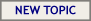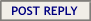Hop to: ContinuousWave: The Whaler GAM or General AreaContinuousWave: Whaler MarketplaceContinuousWave: Whaler Repairs/ModsContinuousWave: Whaler PerformanceContinuousWave: Small Boat ElectricalContinuousWave: Trips and Rendezvous